286
Bit Manipulation
Code Monk
Bit
Bit-fields
Bit-shift-operators
Bit-manipulation
Bitset

Working on bytes, or data types comprising of bytes like ints, floats, doubles or even data structures which stores large amount of bytes is normal for a programmer. In some cases, a programmer needs to go beyond this - that is to say that in a deeper level where the importance of bits is realized.

Operations with bits are used in Data compression (data is compressed by converting it from one representation to another, to reduce the space) ,Exclusive-Or Encryption (an algorithm to encrypt the data for safety issues). In order to encode, decode or compress files we have to extract the data at bit level. Bitwise Operations are faster and closer to the system and sometimes optimize the program to a good level.

We all know that 1 byte comprises of 8 bits and any integer or character can be represented using bits in computers, which we call its binary form(contains only 1 or 0) or in its base 2 form.
Example:
1) 14 = {1110 }2
= 1 * 23 + 1 * 22 + 1 * 21 + 0 * 20
= 14.

2) 20 = {10100 }2
= 1 * 24 + 0 * 23 + 1 * 22 + 0 * 21 + 0 * 20
= 20.

For characters, we use ASCII representation, which are in the form of integers which again can be represented using bits as explained above.

Bitwise Operators:

There are different bitwise operations used in the bit manipulation. These bit operations operate on the individual bits of the bit patterns. Bit operations are fast and can be used in optimizing time complexity. Some common bit operators are:

NOT ( ~ ): Bitwise NOT is an unary operator that flips the bits of the number i.e., if the ith bit is 0, it will change it to 1 and vice versa. Bitwise NOT is nothing but simply the one’s complement of a number. Lets take an example.
N = 5 = (101)2
~N = ~5 = ~(101)2 = (010)2 = 2

AND ( & ): Bitwise AND is a binary operator that operates on two equal-length bit patterns. If both bits in the compared position of the bit patterns are 1, the bit in the resulting bit pattern is 1, otherwise 0.
A = 5 = (101)2 , B = 3 = (011)2 A & B = (101)2 & (011)2= (001)2 = 1

OR ( | ): Bitwise OR is also a binary operator that operates on two equal-length bit patterns, similar to bitwise AND. If both bits in the compared position of the bit patterns are 0, the bit in the resulting bit pattern is 0, otherwise 1.
A = 5 = (101)2 , B = 3 = (011)2
A | B = (101)2 | (011)2 = (111)2 = 7

XOR ( ^ ): Bitwise XOR also takes two equal-length bit patterns. If both bits in the compared position of the bit patterns are 0 or 1, the bit in the resulting bit pattern is 0, otherwise 1.
A = 5 = (101)2 , B = 3 = (011)2
A ^ B = (101)2 ^ (011)2 = (110)2 = 6

Left Shift ( << ): Left shift operator is a binary operator which shift the some number of bits, in the given bit pattern, to the left and append 0 at the end. Left shift is equivalent to multiplying the bit pattern with 2k ( if we are shifting k bits ).
1 << 1 = 2 = 21
1 << 2 = 4 = 22 1 << 3 = 8 = 23
1 << 4 = 16 = 24

1 << n = 2n

Right Shift ( >> ): Right shift operator is a binary operator which shift the some number of bits, in the given bit pattern, to the right and append 1 at the end. Right shift is equivalent to dividing the bit pattern with 2k ( if we are shifting k bits ).
4 >> 1 = 2
6 >> 1 = 3
5 >> 1 = 2
16 >> 4 = 1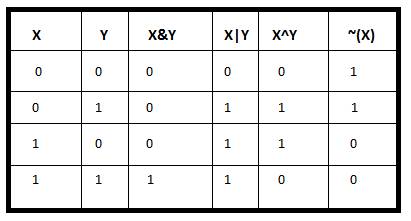Bitwise operators are good for saving space and sometimes to cleverly remove dependencies.

Note: All left and right side taken in this article, are taken with reference to the reader.

Lets discuss some algorithms based on bitwise operations:

1) How to check if a given number is a power of 2 ?
Consider a number N and you need to find if N is a power of 2. Simple solution to this problem is to repeated divide N by 2 if N is even. If we end up with a 1 then N is power of 2, otherwise not. There are a special case also. If N = 0 then it is not a power of 2. Let’s code it.

Implementation:

bool isPowerOfTwo(int x)
{
if(x == 0)
return false;
else
{
while(x % 2 == 0) x /= 2;
return (x == 1);
}
}


Above function will return true if x is a power of 2, otherwise false.
Time complexity of the above code is O(logN).

The same problem can be solved using bit manipulation. Consider a number x that we need to check for being a power for 2. Now think about the binary representation of (x-1). (x-1) will have all the bits same as x, except for the rightmost 1 in x and all the bits to the right of the rightmost 1.
Let, x = 4 = (100)2
x - 1 = 3 = (011)2
Let, x = 6 = (110)2
x - 1 = 5 = (101)2

It might not seem obvious with these examples, but binary representation of (x-1) can be obtained by simply flipping all the bits to the right of rightmost 1 in x and also including the rightmost 1.

Now think about x & (x-1). x & (x-1) will have all the bits equal to the x except for the rightmost 1 in x.
Let, x = 4 = (100)2
x - 1 = 3 = (011)2
x & (x-1) = 4 & 3 = (100)2 & (011)2 = (000)2
Let, x = 6 = (110)2
x - 1 = 5 = (101)2
x & (x-1) = 6 & 5 = (110)2 & (101)2 = (100)2

Properties for numbers which are powers of 2, is that they have one and only one bit set in their binary representation. If the number is neither zero nor a power of two, it will have 1 in more than one place. So if x is a power of 2 then x & (x-1) will be 0.

Implementation:

bool isPowerOfTwo(int x)
{
// x will check if x == 0 and !(x & (x - 1)) will check if x is a power of 2 or not
return (x && !(x & (x - 1)));
}


2) Count the number of ones in the binary representation of the given number.

The basic approach to evaluate the binary form of a number is to traverse on it and count the number of ones. But this approach takes log2N of time in every case.

Why log2N ?
As to get a number in its binary form, we have to divide it by 2, until it gets 0, which will take log2N of time.

With bitwise operations, we can use an algorithm whose running time depends on the number of ones present in the binary form of the given number. This algorithm is much better, as it will reach to logN, only in its worst case.

int count_one (int n)
{
while( n )
{
n = n&(n-1);
count++;
}
return count;
}


Why this algorithm works ?
As explained in the previous algorithm, the relationship between the bits of x and x-1. So as in x-1, the rightmost 1 and bits right to it are flipped, then by performing x&(x-1), and storing it in x, will reduce x to a number containing number of ones(in its binary form) less than the previous state of x, thus increasing the value of count in each iteration.

Example:
n = 23 = {10111}2 .
1. Initially, count = 0.
2. Now, n will change to n&(n-1). As n-1 = 22 = {10110}2 , then n&(n-1) will be {101112 & {10110}2, which will be {10110}2 which is equal to 22. Therefore n will change to 22 and count to 1.
3. As n-1 = 21 = {10101}2 , then n&(n-1) will be {10110}2 & {10101}2, which will be {10100}2 which is equal to 20. Therefore n will change to 20 and count to 2.
4. As n-1 = 19 = {10011}2 , then n&(n-1) will be {10100}2 & {10011}2, which will be {10000}2 which is equal to 16. Therefore n will change to 16 and count to 3.
5. As n-1 = 15 = {01111}2 , then n&(n-1) will be {10000}2 & {01111}2, which will be {00000}2 which is equal to 0. Therefore n will change to 0 and count to 4.
6. As n = 0, the the loop will terminate and gives the result as 4.

Complexity: O(K), where K is the number of ones present in the binary form of the given number.

3) Check if the ith bit is set in the binary form of the given number.

To check if the ith bit is set or not (1 or not), we can use AND operator. How?

Let’s say we have a number N, and to check whether it’s ith bit is set or not, we can AND it with the number 2i . The binary form of 2i contains only ith bit as set (or 1), else every bit is 0 there. When we will AND it with N, and if the ith bit of N is set, then it will return a non zero number (2i to be specific), else 0 will be returned.

Using Left shift operator, we can write 2i as 1 << i . Therefore:

bool check (int N)
{
if( N & (1 << i) )
return true;
else
return false;
}


Example:
Let’s say N = 20 = {10100}2. Now let’s check if it’s 2nd bit is set or not(starting from 0). For that, we have to AND it with 22 = 1<<2 = {100}2 .
{10100} & {100} = {100} = 22 = 4(non-zero number), which means it’s 2nd bit is set.

4) How to generate all the possible subsets of a set ?

A big advantage of bit manipulation is that it can help to iterate over all the subsets of an N-element set. As we all know there are 2N possible subsets of any given set with N elements. What if we represent each element in a subset with a bit. A bit can be either 0 or 1, thus we can use this to denote whether the corresponding element belongs to this given subset or not. So each bit pattern will represent a subset.

Consider a set A of 3 elements.
A = {a, b, c}

Now, we need 3 bits, one bit for each element. 1 represent that the corresponding element is present in the subset, whereas 0 represent the corresponding element is not in the subset. Let’s write all the possible combination of these 3 bits.

0 = (000)2 = {}
1 = (001)2 = {c}
2 = (010)2 = {b}
3 = (011)2 = {b, c}
4 = (100)2 = {a}
5 = (101)2 = {a, c}
6 = (110)2 = {a, b}
7 = (111)2 = {a, b, c}

Pseudo Code:

possibleSubsets(A, N):
for i = 0 to 2^N:
for j = 0 to N:
if jth bit is set in i:
print A[j]
print ‘\n’


Implementation:

void possibleSubsets(char A[], int N)
{
for(int i = 0;i < (1 << N); ++i)
{
for(int j = 0;j < N;++j)
if(i & (1 << j))
cout << A[j] << ‘ ‘;
cout << endl;
}
}


5) Find the largest power of 2 (most significant bit in binary form), which is less than or equal to the given number N.

Idea: Change all the bits which are at the right side of the most significant digit, to 1.

Property: As we know that when all the bits of a number N are 1, then N must be equal to the 2i -1 , where i is the number of bits in N.

Example:
Let’s say binary form of a N is {1111}2 which is equal to 15.
15 = 24-1, where 4 is the number of bits in N.

This property can be used to find the largest power of 2 less than or equal to N. How?
If we somehow, change all the bits which are at right side of the most significant bit of N to 1, then the number will become x + (x-1) = 2 * x -1 , where x is the required answer.
Example:
Let’s say N = 21 = {10101}, here most significant bit is the 4th one. (counting from 0th digit) and so the answer should be 16.
So lets change all the right side bits of the most significant bit to 1. Now the number changes to
{11111} = 31 = 2 * 16 -1 = Y (let’s say).
Now the required answer is (Y+1)>>1 or (Y+1)/2.

Now the question arises here is how can we change all right side bits of most significant bit to 1?

Let’s take the N as 16 bit integer and binary form of N is {1000000000000000}.
Here we have to change all the right side bits to 1.

Initially we will copy that most significant bit to its adjacent right side by:

N = N | (N>>1).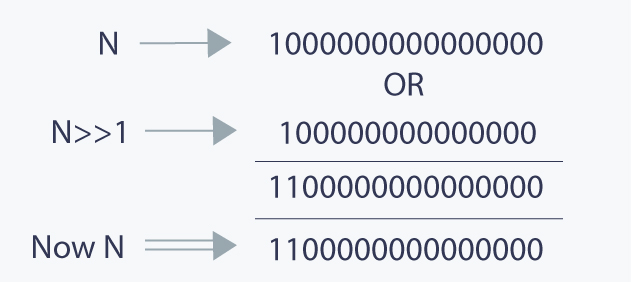As you can see, in above diagram, after performing the operation, rightmost bit has been copied to its adjacent place.

Now we will copy the 2 rightmost set bits to their adjacent right side.
N = N | (N>>2).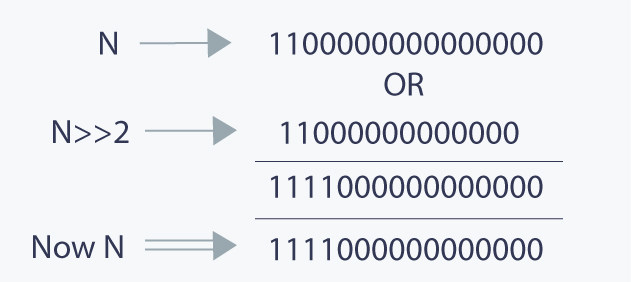Now we will copy the 4 rightmost set bit to their adjacent right side.

N = N | (N>>4)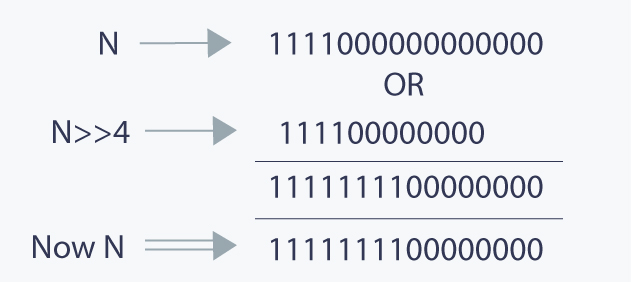Now we will copy these 8 rightmost set bits to their adjacent right side.

N = N| (N>>8)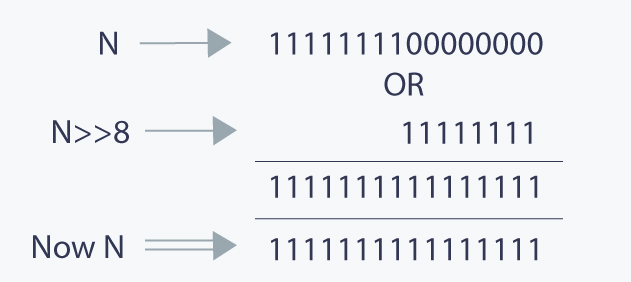Now all the right side bits of the most significant set bit has been changed to 1 .This is how we can change right side bits. This explanation is for 16 bit integer, and it can be extended for 32 or 64 bit integer too.

Implementation:

long largest_power(long N)
{
//changing all right side bits to 1.
N = N| (N>>1);
N = N| (N>>2);
N = N| (N>>4);
N = N| (N>>8);

//as now the number is 2 * x-1, where x is required answer, so adding 1 and dividing it by
2.
return (N+1)>>1;

}


Tricks with Bits:

1) x ^ ( x & (x-1)) : Returns the rightmost 1 in binary representation of x.

As explained above, (x & (x - 1)) will have all the bits equal to the x except for the rightmost 1 in x. So if we do bitwise XOR of x and (x & (x-1)), it will simply return the rightmost 1. Let’s see an example.
x = 10 = (1010)2
x & (x-1) = (1010)2 & (1001)2 = (1000)2
x ^ (x & (x-1)) = (1010)2 ^ (1000)2 = (0010)2

2) x & (-x) : Returns the rightmost 1 in binary representation of x

(-x) is the two’s complement of x. (-x) will be equal to one’s complement of x plus 1.
Therefore (-x) will have all the bits flipped that are on the left of the rightmost 1 in x. So x & (-x) will return rightmost 1.

x = 10 = (1010)2
(-x) = -10 = (0110)2
x & (-x) = (1010)2 & (0110)2 = (0010)2

3) x | (1 << n) : Returns the number x with the nth bit set.

(1 << n) will return a number with only nth bit set. So if we OR it with x it will set the nth bit of x.
x = 10 = (1010)2 n = 2
1 << n = (0100)2
x | (1 << n) = (1010)2 | (0100)2 = (1110)2

Applications of bit operations:

1) They are widely used in areas of graphics ,specially XOR(Exclusive OR) operations.
2) They are widely used in the embedded systems, in situations, where we need to set/clear/toggle just one single bit of a specific register without modifying the other contents. We can do OR/AND/XOR operations with the appropriate mask for the bit position.
3) Data structure like n-bit map can be used to allocate n-size resource pool to represent the current status.
4) Bits are used in networking, framing the packets of numerous bits which is sent to another system generally through any type of serial interface.

To see a creative use of Bitwise operators, you can refer this amazing article , where the bit wise operations are smartly used while developing an online calendar for events.